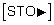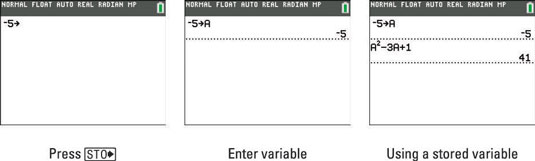##### TI-84 Plus CE Graphing Calculator For DummiesThe letters STO may look like texting language, but the TI-84 Plus calculator's STO key is a handy feature to have around. If you plan to use the same number many times when evaluating arithmetic expressions, consider storing that number in a variable.To do so, follow these steps:

1. If necessary, press [2nd][MODE] to enter the Home screen.

2. Enter the number you want to store in a variable.

You can store a number or an arithmetic expression.

3. PressThe result of this action is shown in the first screen.

4. Press [ALPHA] and press the key corresponding to the letter of the variable in which you want to store the number.

The letters used for storing variables are the letters of the alphabet and the Greek letter theta.

5. Press [ENTER] to store the value.

This is illustrated in the second screen.

## Insert your stored variable into an expression

After you have stored a number in a variable, you can insert that number into an expression. To do so, place the cursor where you want the number to appear, press [ALPHA], and press the key corresponding to the letter of the variable in which the number is stored. See the third screen.The number you store in a variable remains stored in that variable until you or the calculator stores a new number in that variable. Because the calculator uses the letters X, T, and the Greek theta when graphing functions, parametric equations, and polar equations, it is possible that the calculator will change the value stored in these variables when the calculator is in graphing mode.

For example, if you store a number in the variable X and ask the calculator to find the zero of the graphed function X2, the calculator will replace the number stored in X with 0, the zero of X2. So avoid storing values in these three variables if you want that value to remain stored in that variable after you have graphed functions, parametric equations, or polar equations.